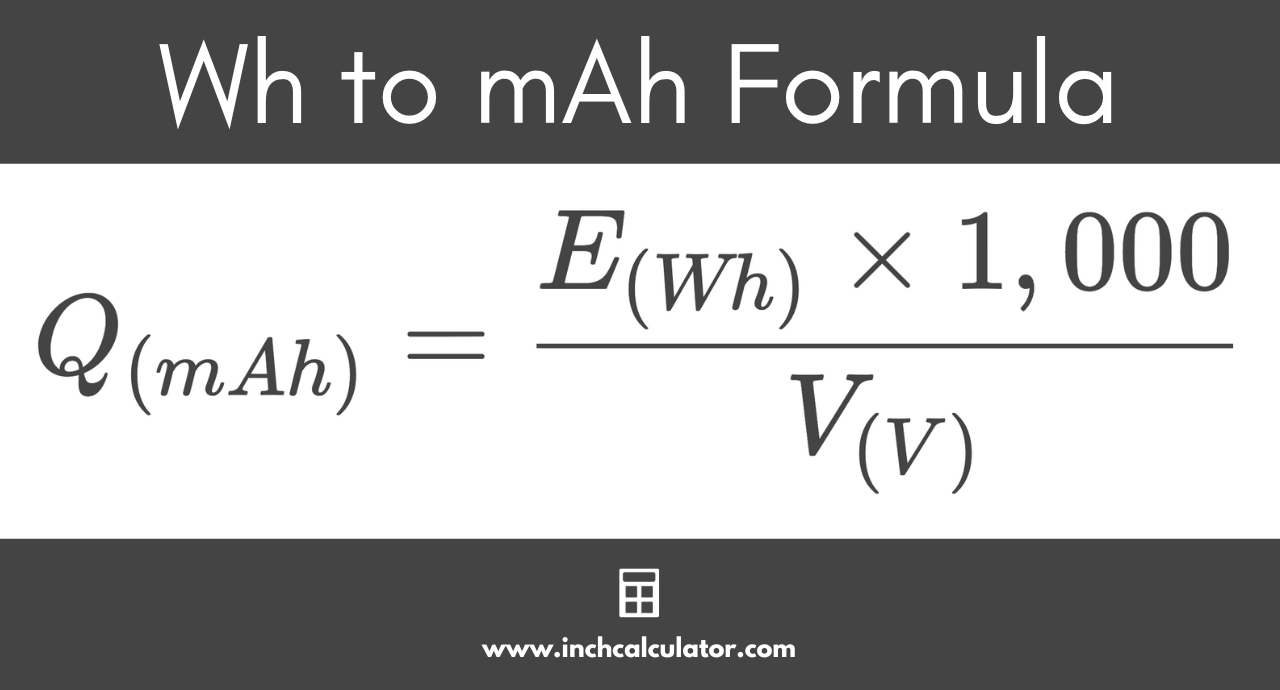# Watt-Hours (Wh) to Milliamp-Hours (mAh) Conversion Calculator

Convert watt-hours to milliamp-hours by entering the energy in watt-hours and the battery terminal voltage below.

Wh
V

## Milliamp-Hours Results:

mAh
Learn how we calculated this below

## How to Convert Watt-Hours to Milliamp-Hours

Units such as watt-hours (Wh) and milliampere-hours (mAh) are often used to describe battery capacity. You may want to convert Wh to mAh, and we’ll go over how to do that below.

Although it is impossible to measure the internal characteristics of a battery because it is a closed system, we can use the observable parameters at the battery’s terminals to estimate internal measurements, such as the stored charge and total energy capacity.

The watt-hour capacity of a battery, expressed as Wh or W·h, is a measure of the amount of energy being supplied to the load. It is a measure of the total electrical power the battery can supply for an hour or the total duration for which it can supply a specific load.

The milliampere-hour capacity of a battery describes the duration for which a battery can supply one milliampere of current and the maximum amount of current it can supply for one hour.

For example, a 10 mAh of capacity means that the battery can supply a load of 10 milliamperes for one hour before losing all its charge, or it can indicate that the battery can supply a 1 milliampere current to a load for 10 hours. The electric charge for smaller batteries is generally measured in milliamp-hours, abbreviated mAh or mA·h.

### Wh to mAh Conversion Formula

To convert from energy to electrical charge, use the formula below in conjunction with the voltage.

Q(mAh) = E(Wh) × 1,000 / V(V)

Thus, the charge in milliamp-hours is equal to the watt-hours times 1,000, then divided by the voltage.You can also convert mAh to Wh using a similar formula.

For example, let’s convert 10 Wh at 12 V to mAh.

Q(mAh) = 10 Wh × 1,000 / 12 V
Q(mAh) = 10,000 mWh / 12 V
Q(mAh) = 833.33 mAh

So, 10 watt-hours of energy at 12 volts is equal to a charge of 833.33 milliamp-hours.

Give our kilowatt-hours to amp-hours calculator a try.